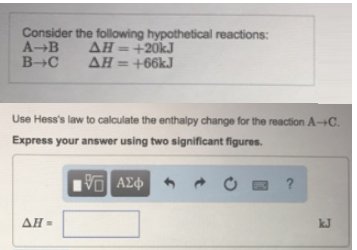# Problem: Consider the following hypothetical reactions:A → B          ΔH = +20kJ B → C         ΔH = +66kJ Use Hess's law to calculate the enthalpy change for the reaction A → C. Express your answer using two significant figures.

###### FREE Expert Solution
83% (213 ratings)###### Problem Details

Consider the following hypothetical reactions:

A → B          ΔH = +20kJ

B → C         ΔH = +66kJ

Use Hess's law to calculate the enthalpy change for the reaction A → C. Express your answer using two significant figures.